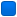Author Topic: How to code a PRNG with discrete uniform distribution?  (Read 3155 times)

Snowman

• GuestHow to code a PRNG with discrete uniform distribution?
« on: April 04, 2015, 12:08:37 pm »
Hello. I want to code a "proper" pseudo-random number generation function.
I'm currently using rand() and modulo (%) to generate values but this is absolutely not the right way!

In C++11 there is std::uniform_int_distribution which is exactly what I would want in C.
Also I have tried reading the Wikipedia article but it did not help me.

In summary: how to code a rand2(limit) function that generates numbers from 0 to limit with a uniform distribution?
Of course, I'm not asking for code and this isn't homework: I would greatly appreciate the formula and a simple explanation.jj2007

• Member
•• Posts: 506Re: How to code a PRNG with discrete uniform distribution?
« Reply #1 on: April 04, 2015, 09:13:14 pm »
Have a look at this site: http://www.cacert.at/cgi-bin/rngresults
Pick a random generator that looks acceptable to you, check if the source or the formula are available, and if yes, start coding. Don't hesitate to ask for advice if you are stuck.

Snowman

• GuestRe: How to code a PRNG with discrete uniform distribution?
« Reply #2 on: April 06, 2015, 11:58:41 am »
Thanks for the link but it left me confused.

I seems to me that I don't really need a PRNG, what I need is an algorithm to trim down the values of existing PRNG to be uniformly distributed. From what I read so far, if I simply do a modulo there will be a distribution bias.

Since I started this thread I've been using Microsoft's CryptGenRandom() instead of rand().

So returning to your link above, what I see are fancy alternatives to CryptGenRandom() which is already good enough, and the trimming problem remains. Please tell me if I miss something, thanks.jj2007

• Member
•• Posts: 506Re: How to code a PRNG with discrete uniform distribution?
« Reply #3 on: April 07, 2015, 07:36:08 pm »
From what I read so far, if I simply do a modulo there will be a distribution bias.

Indeed. I have never understood why the modulo method is so popular among the C/C++ crowd. You get very low bias when multiplying x*(desired range)/(maxrange).

Snowman

• GuestRe: How to code a PRNG with discrete uniform distribution?
« Reply #4 on: April 11, 2015, 03:11:09 pm »
To the StackOverflow topic I previously linked to, someone suggested the function below.
To be honest I don't understand how it works, the code comments seem to be in discordance with the code itself.
It appears to me that the code goes in Undefined Behavior territory.

Code: [Select]
/*
* Calculate a uniformly distributed random number less than upper_bound
* avoiding "modulo bias".
*
* Uniformity is achieved by generating new random numbers until the one
* returned is outside the range [0, 2**32 % upper_bound).  This
* guarantees the selected random number will be inside
* [2**32 % upper_bound, 2**32) which maps back to [0, upper_bound)
* after reduction modulo upper_bound.
*/
u_int32_t
arc4random_uniform(u_int32_t upper_bound)
{
u_int32_t r, min;

if (upper_bound < 2)
return 0;

/* 2**32 % x == (2**32 - x) % x */
min = -upper_bound % upper_bound;

/*
* This could theoretically loop forever but each retry has
* p > 0.5 (worst case, usually far better) of selecting a
* number inside the range we need, so it should rarely need
* to re-roll.
*/
for (;;) {
r = arc4random();
if (r >= min)
break;
}

return r % upper_bound;
}

Additionally, if the function is compiled on Pelles C, a warning about "unary -" pops up.
I've fixed that by using 0-x instead of just -x but I'm concerned I changed the meaning of the code.

Moderators: is there a way to enable syntax highlighting in code?
« Last Edit: April 11, 2015, 03:22:26 pm by Snowman »TimoVJL

• Global Moderator
• Member
••• Posts: 1866Re: How to code a PRNG with discrete uniform distribution?
« Reply #5 on: April 12, 2015, 01:31:15 pm »
is there a way to enable syntax highlighting in code?
Something like this?
Quote
/*
* Calculate a uniformly distributed random number less than upper_bound
* avoiding "modulo bias".
*
* Uniformity is achieved by generating new random numbers until the one
* returned is outside the range [0, 2**32 % upper_bound).  This
* guarantees the selected random number will be inside
* [2**32 % upper_bound, 2**32) which maps back to [0, upper_bound)
* after reduction modulo upper_bound.
*/
u_int32_t
arc4random_uniform(u_int32_t upper_bound)
{
u_int32_t r, min;

if (upper_bound < 2)
return 0;

/* 2**32 % x == (2**32 - x) % x */
min = -upper_bound % upper_bound;

/*
* This could theoretically loop forever but each retry has
* p > 0.5 (worst case, usually far better) of selecting a
* number inside the range we need, so it should rarely need
* to re-roll.
*/
for (;;) {
r = arc4random();
if (r >= min)
break;
}

return r % upper_bound;
}
BTW
There is one for SMF here
« Last Edit: April 13, 2015, 11:55:13 am by TimoVJL »
May the source be with you

Snowman

• GuestRe: How to code a PRNG with discrete uniform distribution?
« Reply #6 on: April 13, 2015, 09:55:21 am »
TimoVJL you are a cheater.Anyway can someone confirm if the posted code does have UB?
I'm also confused about the types of -x versus 0-x when x is unsigned.